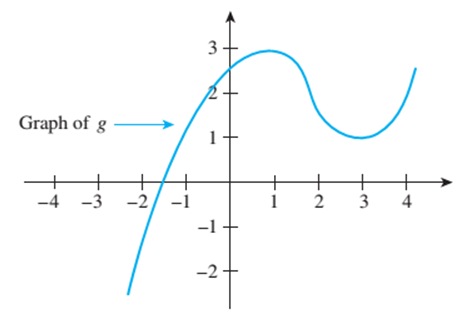Chapter 11.1, Problem 2ES### Discrete Mathematics With Applicat...

5th Edition
EPP + 1 other
ISBN: 9781337694193

#### Solutions

Chapter
Section### Discrete Mathematics With Applicat...

5th Edition
EPP + 1 other
ISBN: 9781337694193
Textbook Problem
1 views

# The graph of a function g is shown below. a. Is g ( 0 ) positive or negative? b Find an approximate value of x so that g ( x ) = 0 . c. Find approximate values for x 1 and x 2 so that g ( x 1 ) = g ( x 2 ) = 1 but x 1 ≠ x 2 . d. Find an approximate value for x such that g ( x ) = − 2 . e. As x increases from − 2 to 1, do the values of g increase or decrease? f. As x increases from 1 to 3, do the values of g increase or decrease?To determine

(a)

Is g(0) positive or negative?

Explanation

Given information:

Concept used:

Value of given function can be determined using graph.

Calculation:

Since

To determine

(b)

Find an approximate value of x so that g(x)=0.

To determine

(c)

Find approximate values for x1 and x2 so that g(x1)=g(x2)=1 but x1x2.

To determine

(d)

Find an approximate value for x such that g(x)=2.

To determine

(e)

As x increases from 2 to 1, do the values of g increase or decrease?

To determine

(f)

As x increases from 1 to 3, do the values of g increase or decrease?

### Still sussing out bartleby?

Check out a sample textbook solution.

See a sample solution

#### The Solution to Your Study Problems

Bartleby provides explanations to thousands of textbook problems written by our experts, many with advanced degrees!

Get Started